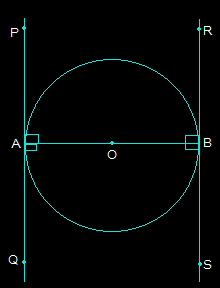Guru

# Prove that the tangents drawn at the ends of a diameter of a circle are parallel. Q.4

• 0

What is the way to solve this question of class 10th of ncert math of circles chapter of exercise 10.2 of math of question no.4, how i prove this question i don’t know how to solve this problem please give me the best and simple way to solve  this problem Prove that the tangents drawn at the ends of a diameter of a circle are parallel.

Share

1. First, draw a circle and connect two points A and B such that AB becomes the diameter of the circle. Now, draw two tangents PQ and RS at points A and B respectively.Now, both radii i.e. AO and OB are perpendicular to the tangents.

So, OB is perpendicular to RS and OA perpendicular to PQ

So, ∠OAP = ∠OAQ = ∠OBR = ∠OBS = 90°

From the above figure, angles OBR and OAQ are alternate interior angles.

Also, ∠OBR = ∠OAQ and ∠OBS = ∠OAP (Since they are also alternate interior angles)

So, it can be said that line PQ and the line RS will be parallel to each other. (Hence Proved).

• 0